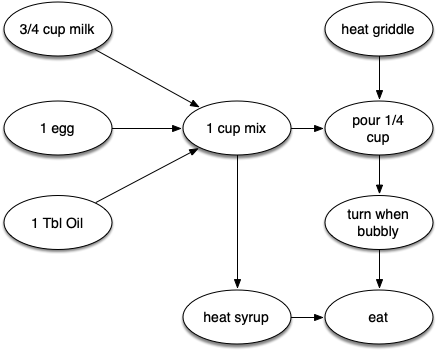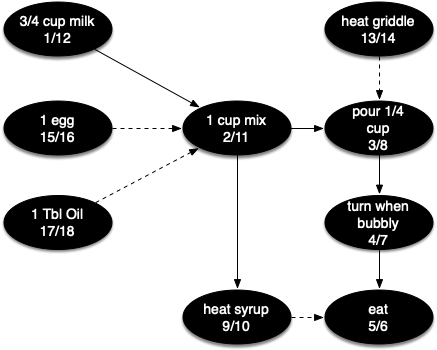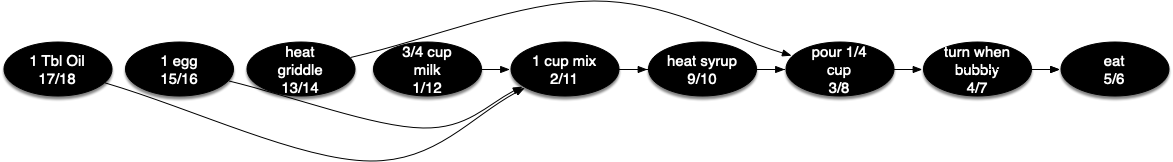8.17. Topological Sorting¶

To demonstrate that computer scientists can turn just about anything into a graph problem, let’s consider the difficult problem of stirring up a batch of pancakes. The recipe is really quite simple: 1 egg, 1 cup of pancake mix, 1 tablespoon oil, and $$3 \over 4$$ cup of milk. To make pancakes you must heat the griddle, mix all the ingredients together and spoon the mix onto a hot griddle. When the pancakes start to bubble you turn them over and let them cook until they are golden brown on the bottom. Before you eat your pancakes you are going to want to heat up some syrup. Figure 27 illustrates this process as a graph.Figure 27: The Steps for Making Pancakes

The difficult thing about making pancakes is knowing what to do first. As you can see from Figure 27 you might start by heating the griddle or by adding any of the ingredients to the pancake mix. To help us decide the precise order in which we should do each of the steps required to make our pancakes we turn to a graph algorithm called the topological sort.

A topological sort takes a directed acyclic graph and produces a linear ordering of all its vertices such that if the graph $$G$$ contains an edge $$(v,w)$$ then the vertex $$v$$ comes before the vertex $$w$$ in the ordering. Directed acyclic graphs are used in many applications to indicate the precedence of events. Making pancakes is just one example; other examples include software project schedules, precedence charts for optimizing database queries, and multiplying matrices.

The topological sort is a simple but useful adaptation of a depth first search. The algorithm for the topological sort is as follows:

1. Call dfs(g) for some graph g. The main reason we want to call depth first search is to compute the finish times for each of the vertices.

2. Store the vertices in a list in decreasing order of finish time.

3. Return the ordered list as the result of the topological sort.

Figure 28 shows the depth first forest constructed by dfs on the pancake-making graph shown in Figure 26.Figure 28: Result of Depth First Search on the Pancake Graph

Finally, Figure 29 shows the results of applying the topological sort algorithm to our graph. Now all the ambiguity has been removed and we know exactly the order in which to perform the pancake making steps.Figure 29: Result of Topological Sort on Directed Acyclic Graph